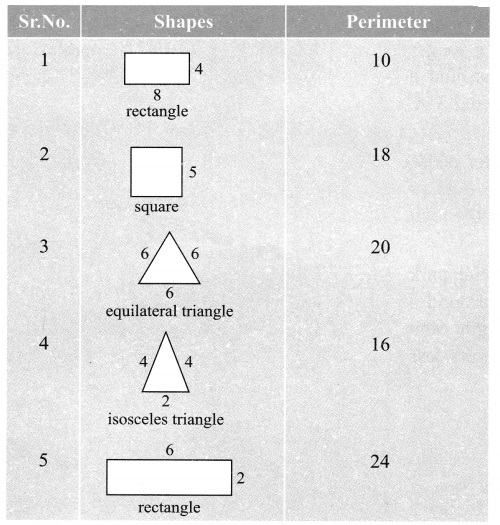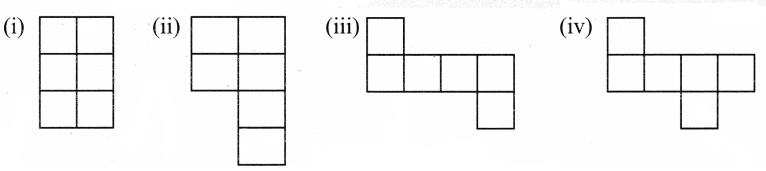## Grade 6 Maths Mensuration Multiple Choice Questions (MCQs)

1. The length and breadth of a rectangle are 40 cm and 10 cm respectively. Its perimeter is:
(a) 100 cm
(b) 120 cm
(c) 140 cm
(d) none of these

2. The side of a square is 8 cm. Its area is:
(a) 64 cm2
(b) 84 cm2
(c) 100 cm2
(d) none of these

3. The area of a rectangle is 40 cm2. If its breadth is 4 cm, then its length is:
(a) 20 cm
(b) 30 cm
(c) 10 cm
(d) none of these

4. The area of square is 100 cm2. Its side is:
(a) 20 cm
(b) 30 cm
(c) 10 cm
(d) none of these

5. The side of a square is 12 m. Its perimeter is:
(a) 36 m
(b) 40 m
(c) 42 m
(d) none of these

6. If the area of a square is 2.25 m2, then its perimeter is:
(a) 7 m
(b) 5 m
(c) 6 m
(d) none of these

7. The side of a square is 8 cm. If its side is doubled, then its new perimeter is:
(a) 64 cm
(b) 81 cm
(c) 121 cm
(d) none of these

8. The length and breadth of a rectangle are 10 cm and 8 cm respectively. If its length is doubled, then its new area is:
(a) 80 cm2
(b) 160 cm2
(c) 240 cm2
(d) none of these

9. The amount of surface enclosed by a closed figure is called its:
(a) perimeter
(b) area
(c) flat surface
(d) interior region

10. What is the perimeter of a regular pentagon whose each side measuring 5 cm?
(a) 10 cm
(b) 20 cm
(c) 15 cm
(d) 25 cm

11. What will be the cost of tilting a rectangular plot of area 800 sq.m, if the cost of tiling 100 sq.m is ₹ 6
(a) ₹ 14
(b) ₹ 48
(c) ₹ 4800
(d) ₹ 900

12. What is the length of the garden if area of rectangular garden of width 60 m is 300 sq.m?
(a) 900 m
(b) 90 m
(c) 18 m
(d) 5 m

13. The perimeter of a triangle whose sides are 5 cm, 2 cm and 3 cm.
(a) 30 cm
(b) 11 cm
(c) 17 cm
(d) 10 cm

14. What is the length of side of square whose area is 64 m2?
(a) 16 m
(b) 32 m
(c) 8 m
(d) 64 m

15. The perimeter of an isosceles triangle with equal sides of length 4 cm and third side of length 6 cm will be:
(a) 10 cm
(b) 8 cm
(c) 20 cm
(d) 14 cm

16. The perimeter of a rectangle is 130 m. If the breadth of the rectangle is 30 m, find its area.
(a) 640 m2
(b) 600 m2
(c) 700 m2
(d) none of these

17. The sides of a rectangle are in the ratio 5 : 4. If its perimeter is 72 cm then the length is:
(a) 20 cm
(b) 30 cm
(c) 40 cm
(d) none of these

18. The cost of putting a fence around a square field at ₹ 2.50 per metre is ₹ 200. The length of each side of the field is:
(a) 40 m
(b) 20 m
(c) 80 m
(d) none of these

19. The area of a rectangle is 650 cm2 and one of its sides is 13 cm. Find the perimeter of the rectangle.
(a) 120 cm
(b) 130 cm
(c) 126 cm
(d) none of these

20. A room is 5m 40cm long and 3m 75cm wide. Find the area of the carpet needed to cover the floor.
(a) 20 m2
(b) 20.25 m2
(c) 21 m2
(d) none of these

### Class 6 Maths Mensuration Match the following### Class 6 Maths Mensuration Very Short Answer Type Questions

1. Find the perimeter of equilateral triangle whose each side is 7cm.
2. Find the length of a rectangle whose perimeter is 52 cm and breadth is 12 cm.
3. A rectangular carpet measures 3m 45cm by 2m 25cm . What is perimeter of the carpet?
4. Leena bent a wire 132cm long into a square. What is the length of the side of a square?
5. Find the perimeter of a triangle whose sides are 5cm, 7cm and 10cm.
6. Find the perimeter of a regular pentagon with each side measuring 7cm.

### Class 6 Maths Mensuration Short Answer Type Questions

1. Find the cost of fencing a rectangular park of length 450m and breadth 300m at the rate of ₹ 32 per meter.
2. How much distance does a jooger cover if he runs 5 times around a rectangular Park 76m long and 35m wide?
3. A rope costing ₹ 8 per meter needs to be laid around a square field of side 150m. How much will the total rope cost?
4. Sweety runs around a square park of side 75m. Bulbul runs around a rectangular park with length 60m and breadth 45m. Who covers less distance?

### Class 6 Maths Mensuration Long Answer Type Questions

1. How much would it cost to lay a wall to wall carpet in a room 10m long and 7m wide, with a carpet that costs ₹ 115 per m2.
2. How many tiles of 10cm by 6cm will be needed to pave a rectangular path of 5m by 3m?
3. Following figures are formed by joining six unit squares. Which figure has the smallest perimeter?